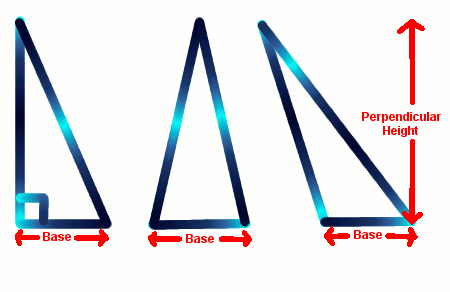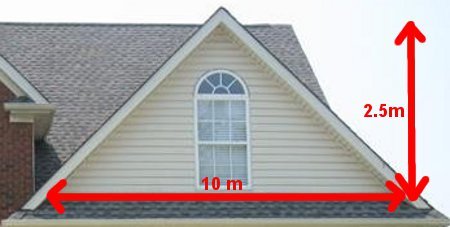# Area Of Triangle

If you fold a square piece of paper diagonally (from corner to corner) in half, it makes a triangle.

If you fold a rectangular piece of paper diagonally in half, it makes another triangle.

Therefore, the rule for the Area of a Rectangle and the Area of a Triangle are related.Area of Triangle = B × H 2

where
B is the base
H is the perpendicular height (at right angles to the base).

All 3 triangles above have the same base, the same perpendicular height and therefore, all 3 triangles have the same area.

## Example - Painting a Roof GableIn this diagram of a roof gable at the front of a house, what is the area to be painted? (Include the window so there's paint left over.)

Area of triangle
= (B × H) ÷ 2
= 10 × 2.5
= 25 m2

## Maths Fun - Heron's Formula

Though more complex, this is a useful rule in real life because we only need to know the lengths of the 3 sides of any shaped triangle to find the area.

The sides are called a, b, and c.

Heron's rule has 2 parts:

 S = (a + b + c) 2

Area of triangle = √S ( S – a) (S – b) (S – c)

What is the area of a triangular city park whose sides are 100 metres, 150 metres and 200 metres?